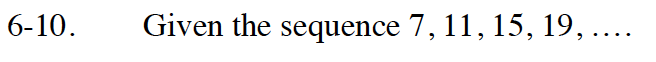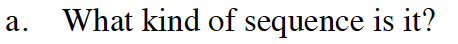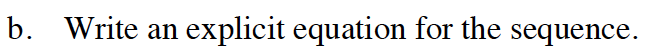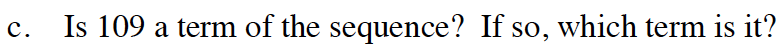Home > GB8I > Chapter cc46 > Lesson cc46.1.1 > Problem6-10

6-10.
1. Given the sequence 7, 11, 15, 19, …. Homework Help ✎

1. What kind of sequence is it?

2. Write an explicit equation for the sequence.

3. Is 109 a term of the sequence? If so, which term is it?How do you get from one term to the next?
By adding or by multiplying?See the online help for problem 5-68. Remember that t(n) = mn + t(0).Let t(n) = 109 in the equation you wrote for part (b).
Solve for n. Remember that n is the term number.

n = 26.5, so no, 109 is not a term in the sequence.